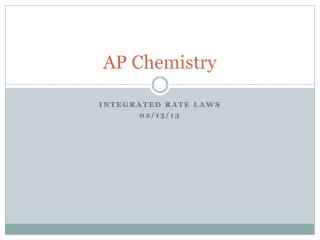DownloadDownload PresentationAP Chemistry

# AP Chemistry

Télécharger la présentation## AP Chemistry

- - - - - - - - - - - - - - - - - - - - - - - - - - - E N D - - - - - - - - - - - - - - - - - - - - - - - - - - -
##### Presentation Transcript

1. AP Chemistry Integrated Rate Laws 02/13/13

2. Integrated vs. Differential Rate Laws Differential Rate Law: A rate law that expresses how the rate depends on the concentration of the reactants. (Often simply called “a rate law.”) Integrated Rate Law: A rate law that expresses how the concentrations depend on time.

3. First Order Rate Laws Consider the reaction: aA products The rate is -∆[A]/ ∆t = k[A]n Since it is often useful to consider the change in concentration in terms of time, the following law is derived from the previous equation by some sort of miracle. ln[A] = -kt + ln[A]0 ln = natural log, k = rate constant, t = time, [A] is at time t, [A]0 is at time 0 (the start of the experiment).

4. ln[A] = -kt + ln[A]0 This equation shows how the concentration of A depends on time. If the initial concentration and k are known, the concentration of A at any time can be calculated.

5. ln[A] = -kt + ln[A]0 Note that the form of this equation is y = mx + b. y = ln[A] x = t m = -k b = ln[A]0 Therefore, plotting the natural log of concentration vs. time will always produce a straight line for a first order reaction. If the plot is a straight line, the reaction is first order; if the plot is not a straight line, the reaction is not first order.

6. ln[A] = -kt + ln[A]0 The integrated rate law can also be expressed in terms of a ratio of [A] and [A]0. ln([A]0/A]) = kt

7. First Order Rate Laws Consider 2N2O5(g)  4 NO2(g) + O2(g) Using this data, verify that the rate law is first order and calculate k.

8. -2 -4 -6 100 200 300 400

9. First Order Rate Laws Since the reaction is first order, then the slope of the line is equal to -k = ∆y/ ∆x = ∆(ln[N2O5])/ ∆t Since the first and last points are exactly on the line, we will use them to calculate slope. k = -(slope) = 6.93 x 10-3 s-1

10. First Order Rate Law Using the previously obtained data, calculate [N2O5] at 150 seconds after the start of the reaction.

11. Half Life of a First Order Reaction Half Life: the time required for a reactant to reach half its original concentration. ln([A]0/A]) = kt By definition at t =½: [A] = [A]0/2 ln(2) = kt½ t½ = 0.693/k

12. Half Life for First Order Reaction A certain first order reaction has a half-life of 20.0 minutes. Calculate the rate constant for this reaction. How much time is required for this reaction to be 75% complete? ln([A]0/A]) = kt

13. The decomposition of A is first order, and [A] is monitored. The following data are recorded: Calculatethe rate constant. Calculate the half life. Calculate [A] when t = 5 min.How long will it take for 90% of A to decompose?

14. Reaction Rate: Concentration NH4+(aq) + NO2-(aq)  N2(g) + 2H2O(l)

15. The reaction 2ClO2(aq) + 2OH-(aq)ClO3-(aq) + ClO2-(aq) + H2O (l) was studied with the following results. Exp[ClO2][OH-]Rate (M/s) 1 0.060 0.030 0.0248 2 0.020 0.030 0.00276 3 0.020 0.090 0.00828 (a) Determine the rate law (b) Calculate the value of the rate law constant